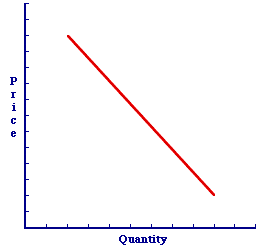Sunday  September 25, 2022
 AmosWEB means Economics with a Touch of Whimsy!INFLATION RATE: The percentage change in the price level from one period to the next. The two most common price indices used to measure the inflation are the Consumer Price Index (CPI) and the GDP price deflator.CHANGE IN QUANTITY DEMANDED:

A movement along a given demand curve caused by a change in demand price. The only factor that can cause a change in quantity demanded is price. A related, but distinct, concept is a change in demand.
A change in quantity demanded is a change in the specific quantity of a good that buyers are willing and able to buy. This change in quantity demanded is caused by a change in the demand price. It is illustrated by a movement along a given demand curve.

In fact, the only way to induce a change in quantity demanded is with a change in the price. Anything else, everything else, causes a change in demand.

As the demand price induces a change in the quantity demanded and a movement along the demand curve, the five demand determinants (buyers' income, buyers' preferences, other prices, buyers' expectations, and number of buyers) remain unchanged.

### Demand and Quantity Demanded

To set the stage for an understanding of this difference, take note of two related concepts:
• Quantity Demanded: Quantity demand is a specific quantity that buyers are willing and able to buy at a specific demand price. It is but ONE point on a demand curve.

• Demand: Demand is the range of quantities that buyers are willing and able to buy at a range of demand prices. It is ALL points that make up a demand curve.

### Making Changes

So what happens when the phrase "change in" is placed in front of each term?
• Change in Quantity Demanded: A change in quantity demanded is a change from one price-quantity pair on an existing demand curve to a new price-quantity pair on the SAME demand curve. In other words, this is a movement along the demand curve. A change in quantity demanded is caused by a change in price.

• Change in Demand: A change in demand is a change in the ENTIRE demand relation. This means changing, moving, and shifting the entire demand curve. The entire set of prices and quantities is changing. In other words, this is a shift of the demand curve. A change in demand is caused by a change in the five demand determinants.

### Changing the Quantity

A Change in Quantity DemandedA change in quantity demanded is a movement along a given demand curve. A change in demand is a shift of the demand curve. These alternatives can be illustrated with the negatively-sloped demand curve presented in this exhibit. This demand curve captures the specific one-to-one, law of demand relation between demand price and quantity demanded. The five demand determinants are assumed to remain constant with the construction of this demand curve.
• A Change in Quantity Demanded: A change in quantity demanded, which is only triggered by a change in demand price, is a movement along the demand curve. Click the [A Price Change] button to demonstrate.

• A Change in Demand
: A change in demand, which is triggered by a change in any of the five demand determinants, is a shift of the demand curve. Click the [A Determinant Change] button to demonstrate.

### An Important Difference

Why is this difference so important? The answer is as simple as cause and effect. The demand curve is used (together with supply) to explain and analyze market exchanges. The sequence of events follows a particular pattern.
• First, a demand (or supply) determinant changes.

• Second, this determinant change causes the demand curve (or supply curve) to shift.

• Third, the change in demand (or supply) causes either a shortage or a surplus imbalance in the market. The market is in a temporary state of disequilibrium.

• Fourth, the shortage and surplus imbalance causes the price of the good to change.

• Fifth, the change in price causes a change in quantity demanded (and supplied).

• Sixth, the change in quantity demanded (and supplied) eliminates the shortage or surplus and restores market equilibrium.

The key conclusion is that demand (and supply) determinants, which induce changes in demand (and supply), are the source of instability in the market. The change in price, which induces a change in quantity demanded (and supplied) is the means of eliminating the instability and restoring equilibrium.

 <= CHANGE IN PRIVATE INVENTORIES CHANGE IN QUANTITY SUPPLIED =>Recommended Citation:

CHANGE IN QUANTITY DEMANDED, AmosWEB Encyclonomic WEB*pedia, http://www.AmosWEB.com, AmosWEB LLC, 2000-2022. [Accessed: September 25, 2022].

Check Out These Related Terms...

Or For A Little Background...

And For Further Study...
Search Again?ORANGE REBELOON[What's This?] Today, you are likely to spend a great deal of time at an auction wanting to buy either a flower arrangement for that special day for your mother or a New York Yankees baseball cap. Be on the lookout for rusty deck screws.Your Complete ScopeOn a typical day, the United States Mint produces over \$1 million worth of dimes."If I'm selecting a group, the first thing I look for is a record of achievement . . . If (candidates achieve) in small things, there's a very good chance they'll perform well in big things. "-- Edmund Hillary, explorerDDemandA PEDestrian's Guide Xtra CreditTell us what you think about AmosWEB. Like what you see? Have suggestions for improvements? Let us know. Click the User Feedback link.| | | | | | | | | | |
| | | |

Thanks for visiting AmosWEB